On my last post,i discussed about why we need to determine motor loads and efficiency.So for this post i want to share how to get the motor load value using simple calculation electrical formula.

Before we go further,i want to explain about Power.What is meaning of Power?Of course this term is significant with our discussion topic.Power is rated of energy in electrical circuit.

It is combination from Amperage and Voltage value.Basic formula for Power is P (watt) =I(ampere)  x V (volt).Power also measured in Horsepower (hp) unit.For common conversion from electrical Horsepower to Watt is 1hp = 746 watt.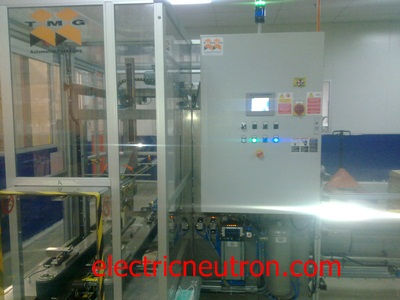## How to calculate Motor Loads

Electrical motor loads are calculated from power in kW and full rated load in kW.Following is the formula how to calculate Motor loads.

Example :

We have 1 unit Induction Motor with 30 horsepower (hp),running with 34.9 amps for amperage load and 460 volts 3 phase  and power factor value is 0.75 and motor efficiency is 85%

STEP 1

We determine value of power in kW (Pi) using this formula.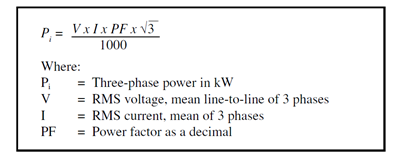STEP 2

We need to determine the full rated load in kW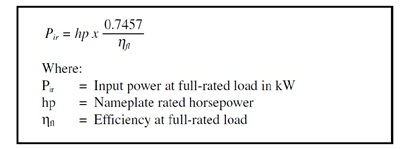STEP 3

We can quantify the motor’s part-load by comparing the measured input power under load to the power required when the motor operates at rated capacity using this formula.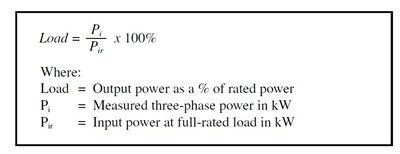Calculation :-

First step for actual value of voltage and ampere,i suggest to collected measurement data from motor using the Voltmeter and Clamp on meter.Example as following :-

a) VOLTAGE

V red = 462 volt    V blue = 461 volt    V yellow = 460 volt

V total = (462 + 461 + 460) / 3 = 461 volt

b) AMPERE

A red = 34.5 amp   A blue = 33.8 amp   A yellow = 34.2 amp

A total = (34.5+33.8+34.2) / 3 = 34.1 amp

c) Power Factor : 0.75

d) Motor efficiency : 85%

Formula 1 :

Pi = (V xI x PF x 1.732) / 1000

Pi =  (461 x 34.1 x 0.75 x 1.732) / 1000

Pi = 20.4 kW

Formula 2

Pir = (HP x 0.7457)/Efficiency

Pir = ( 30 x 0.7457) / 0.85

Pir = 19 kW

Formula 3

LOAD = (Pi / Pir) x 100%

Output power as a % of rated power = 107.4%

* From this example value,we can determine our electric motor load in good condition.It is 100% with output power to drive the equipment.It efficiency and not to run with overload.

1.PABITRA MAITY says

how can I found out operational efficiency of a motor?

2.Su Lim says

If i am going to use the motor with high and low load alternating in very fast time (less than 1 second), but i don’t know how to measure the load, but i can measure the current drawn. how do i calculated motor load for me to select the right size of motor?

3.dhiraj bhosale says

Plz tell me how to calculate total load to my box manufacturing plant
My machines specification as follows
Currogation machine 5hp
Pasting machine 3hp
Stitching machine 2 nos 0.5 hp
Slotting machine 3hp

•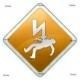Lemau says

Hi,

P = I x V x 1.732 –> 3 phase power supply

P= Power (watt)
I = Ampere
V= Voltage

•win says

(Output power as a % of rated power = 107.4% )
i really don’t understand the above result.
output power is higher than input power?

•jake says

yeah as i know output power should be smaller than input power

•shaik Abdul Umraj says

In above formula 2
pir = 26.31
load (pi / pir) x 100% = 77.53%

output power as a% of rated power = 77.53%

•vael says

Very easy calculate from amps each unit and total it get answer. I beleive you know each unit kw which u state horsepower only.

4.dhiraj bhosale says

Board cutting machine 3 hp

5.John milton says

I made a blower the fan Wight 55Kg , OD-33.5″, width -6″
So which capecity motor required
And till me formula of this plzz

6.Duncan says

In what universe is this true:

Formula 2

Pir = (HP x 0.7457)/Efficiency

Pir = ( 30 x 0.7457) / 0.85

Pir = 19 kW

Nice going algebra boy, but it is a multiplied inefficiency not a divided.

HP * 746 WATTS / HP * LOSS = HP KW
HP KW / VOLTAGE = FULL LOAD AMPS

Hence,
30 HP with a 0.85 Efficiency = 19 KW

Divide by the Voltage measured or supplied and get Amps, so in this example with 460 the FLA should be 41.35

•shaik Abdul Umraj says

pir = 26.31

7.Fida says

Formula 2

Pir = (HP x 0.7457)/Efficiency

Pir = ( 30 x 0.7457) / 0.85

Pir = 19 kW
The above calculation is not correct, the answer should 26.318 KW

•shaik Abdul Umraj says

yes,

output power as a% of rated power = 77.53%

8.Sanu kumar sahu says

How to know the d.c motor and a.c motor full load calculation formula ???

9.Max says

Hi
I’ve got a 260 KW motor on a soft starter also rated at 260KW it was originally connected to a 330KW gearbox and pump after many years the gearbox failed and can only get a 409KW gearbox to meet my specs will my motor and soft starter handle the load of the gear box I know I’ll loose efficiency but will I lose my motor and starter??

10.shaik Abdul Umraj says

which method is correct for measuring electrical load of motors?
Ampere load by amp clamp meter or KW load by KW clamp meter?

11.Akhilesh Yadav says

I have used 132 Kw( 227 amp & 415 V) / 4 pole motor with to a fan drawing 85.5 Amp & 227 V at 1020 rpm, What is the power input to motor

This site uses Akismet to reduce spam. Learn how your comment data is processed.